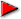ChaptersPage 3This text is meant to accompany class discussions. It is not everything there is to know about the basics of electricity. It is meant as a  prep for class. More detailed notes and examples are given in the class notes, presentations, and demonstrations (click here.)

Ohm's Law

If you change the voltage through a piece of electronics, such as a wire, while measuring the current through the wire you may get a graph like the one below.Georg Simon Ohm noticed this relationship and called the relationship "Ohm's Law." In this graph The voltage divided by the current gives a constant slope. This slope is called the resistance. This means thatWhere ΔV is the difference in voltage.

Any device that behaves like the graph above (with a straight line) is called an ohmic device. Many electrical components in today's electronics do not follow Ohm's law. But everything we will study does follow Ohms law. Examples of ohmic devices would be a wire, incandescent light bulb, electric stove heating element or a resistor. Electronic appliances like music players, computers, telephones, etc are NOT ohmic devices.

 Example An electric fan has a resistance of 100 Ω and is plugged into a wall outlet. How much current runs through the fan? Click to here to see an image of the answer without the video.

Voltage and "Potential Difference"

The ball at the bottom of each ramp on the table top has the same speed as the ball at the bottom of the ramp on the floor. The speed of the ball is not dictated by the where the ramp is placed but only the difference in height between the starting point and the ending point. The "gravitational potential" energy is the same for each ball.

Voltage works the same way. To operate a flashlight, radio, iPod, lamp television, etcetera, it takes a difference in energy, ΔV. This difference in electric potential energy is referred to as "potential difference." It is another word for what we have been calling voltage.

Remember the MP3 player in the beginning?

The charges lost some of there energy to the MP3 player. Not all of it. It is the difference in energy that made the player work. That was the "potential difference" that made the player work.

Types of currents

The flow of charges out of a battery is constant like the flow of water in a river. If this flow is measured over time, then is would constant.This constant flow or current is called a direct current. "d.c." for short.Typically d.c. sources come from batteries.However, there are other d.c. sources. Like the power supplies in computers and a.c. adapters. (But some cheaper a.c. adapters have a current with a small wiggle.)If the flow in a river in a river could travel back and forth, then some of the time the flow would go forwards and and then backwards. At times it would even stop for a moment. This type of current is called an alternating current because is direction alternates. As a function of time, it would look like this graph.This type of current comes from a wall outlet.Example A fan is plugged into a wall outlet. The fan draws 0.50 Amps from the outlet. How much resistance is in the fan's motor? [ Anything plugged into a wall is assumed to have a voltage of 120 Volts. (This is an implied given you need to know.) ]

by Tony Wayne ...(If you are a teacher, please feel free to use these resources in your teaching.)